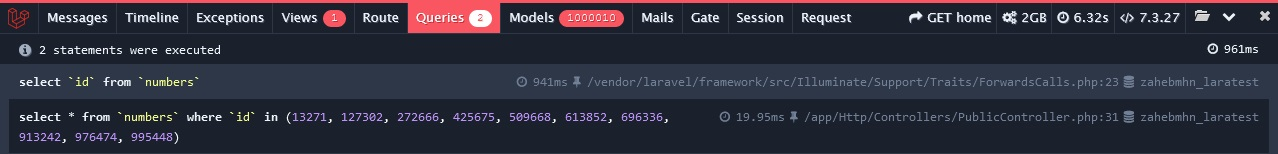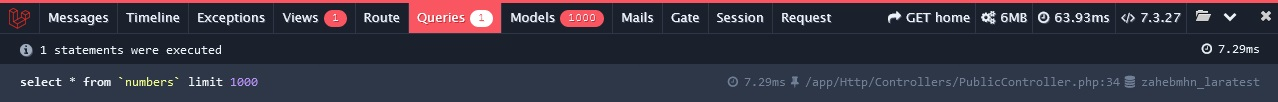Select Page

Sometimes we want to show random data from a table. Eg.

• Showing 10 random blog posts from 1000s of posts.
• Showing 10 random questions from 100s of questions in a Quiz.
• Showing 10 random phone numbers from millions of phone numbers.

I’m going to share a few good ways, that I know. But there could be many other better ways to do it.

## Using inRandomOrder() method :

Laravel >= 5.2 have inRandomOrder() method to take random records from a database table, and it’s quite simple.

``````\$numbers = DB::table('numbers')->inRandomOrder()->take(10)->get();
// OR using Model
\$numbers = Number::inRandomOrder()->take(10)->get();``````

But, the bad thing about this method is, if you’ve got a large table, eg. 500K or 1M or more rows, it will take a lot of time to retrieve the records from database table compared to other methods that I’ll show below.## Using PRIMARY KEY column :

First, you run a query to take all values of the primary key column. Then, take 10 random values from them. Finally, run another query to take rows from the table using the 10 random values as whereIn() values. This method would be a bit faster than the previous method.

``````\$numbers = DB::table('numbers')->get('id')->random(10);
\$numbers_id = Arr::pluck(\$numbers, 'id');
\$numbers = DB::table('numbers')->whereIn('id', \$numbers_id)->get();
// OR using Model
\$numbers = Number::get('id')->random(10);
\$numbers_id = Arr::pluck(\$numbers, 'id');
\$numbers = Number::whereIn('id', \$numbers_id)->get();``````## Using take() and random() method :

If you have a large table, you can take recent 1000 (any good large amount) rows using the take() method, then take 10 random rows using the random() method. Using this method, you’ll get the random rows faster.

``````\$numbers = DB::table('numbers')->take(1000)->get()->random(10);
// OR using Model
\$numbers = Number::take(1000)->get()->random(10);``````## Using a sorting column and CRON job :

Create an additional column, name it something like – ‘sorter’. Create a CRON Job or Scheduled Task to set/update this column’s values between 1-100 integers every few hours. Then when you run a query, sort the query by that column. This method could be faster, but a bit slower than the previous method to take random records from a table.

``````\$numbers = DB::table('numbers')->orderBy('sorter')->take(10)->get();
// OR using Model
\$numbers = Number::orderBy('sorter')->take(10)->get();``````To test the above methods, I’ve used a table named “numbers” with 1M phone numbers in it. So, If you have a table with such an amount of data, use the right method to get random data.